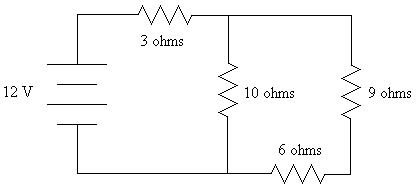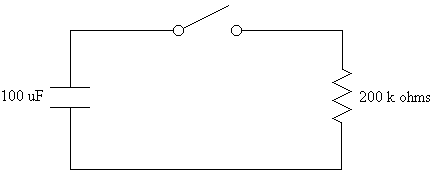First Name: ___________________ Last Name: ____________________ Section: _________

February 19, 1999 Physics 208

Exam 1

Print your name and section clearly on all five pages. (If you do not know your section number, write your TAs name.) Show all work in the space immediately below each problem. Your final answer must be placed in the box provided. Problems will be graded on reasoning and intermediate steps as well as on the final answer. Be sure to include units wherever necessary, and the direction of vectors. Each problem is worth 25 points. In doing the problems, try to be neat. Check your answers to see that they have the correct dimensions (units) and are the right order of magnitudes. You are allowed one 8½ x 11" sheet of notes and no other references. The exam lasts exactly 50 minutes.

(Do not write below)

SCORE:

Problem 1: __________

Problem 2: __________

Problem 3: __________

Problem 4: __________

TOTAL: ___________

First Name: ___________________ Last Name: ____________________ Section: _________

1. A solid, non-conducting ball with a radius of 30 cm has a charge of 2 µC distributed uniformly throughout its interior.
a. Calculate the electric field at a distance of 10 cm from the center of the ball. (10 pts.)

b. Calculate the potential difference between the center of the ball and its surface. (8 pts.)

c. Calculate the force on a 1-µC charge just outside the ball. (7 pts.)

First Name: ___________________ Last Name: ____________________ Section: _________

1. In the circuit below, the battery can supply unlimited current, and the resistors obey Ohms law.a. Calculate the voltage drop across the 6-ohm resistor. (15 pts.)

b. Calculate the electrical power produced by the battery. (10 pts.)

First Name: ___________________ Last Name: ____________________ Section: _________

1. In the circuit below, the capacitor is initially charged to 50 V, and then the switch is closed.a. What energy is stored in the capacitor before the switch is closed? (6 pts.)

b. What power is dissipated by the resistor just after the switch is closed? (7 pts.)

c. What voltage is across the capacitor 1 minute after the switch is closed? (6 pts.)

d. What charge flowed out of the capacitor during the first minute after the switch was closed? (6 pts.)

First Name: ___________________ Last Name: ____________________ Section: _________

4. An electron (q = -1.6 x 10-19 C, m = 9.1 x 10-31 kg) is accelerated from rest through a potential difference of 20 kV and then enters a region of space where the magnetic field is 200 G perpendicular to the direction of the electrons motion.

a. How far into the magnetic field does the electron penetrate? (10 pts.)

b. How long does it take for the electron to exit the field after it enters? (10 pts.)

c. If 1016 such electrons are accelerated in this way each second, what electrical power is consumed by the accelerator? (5 pts.)